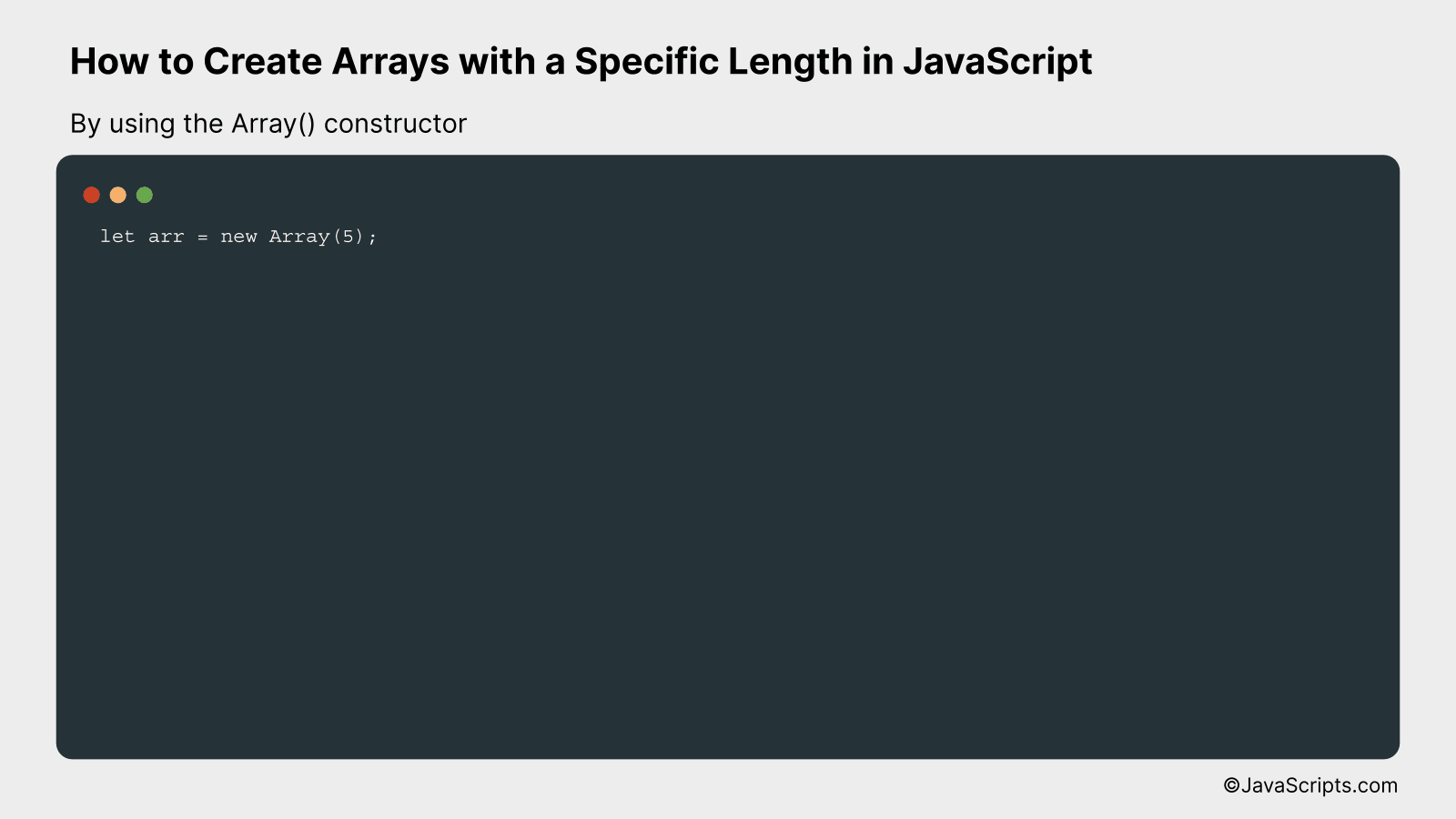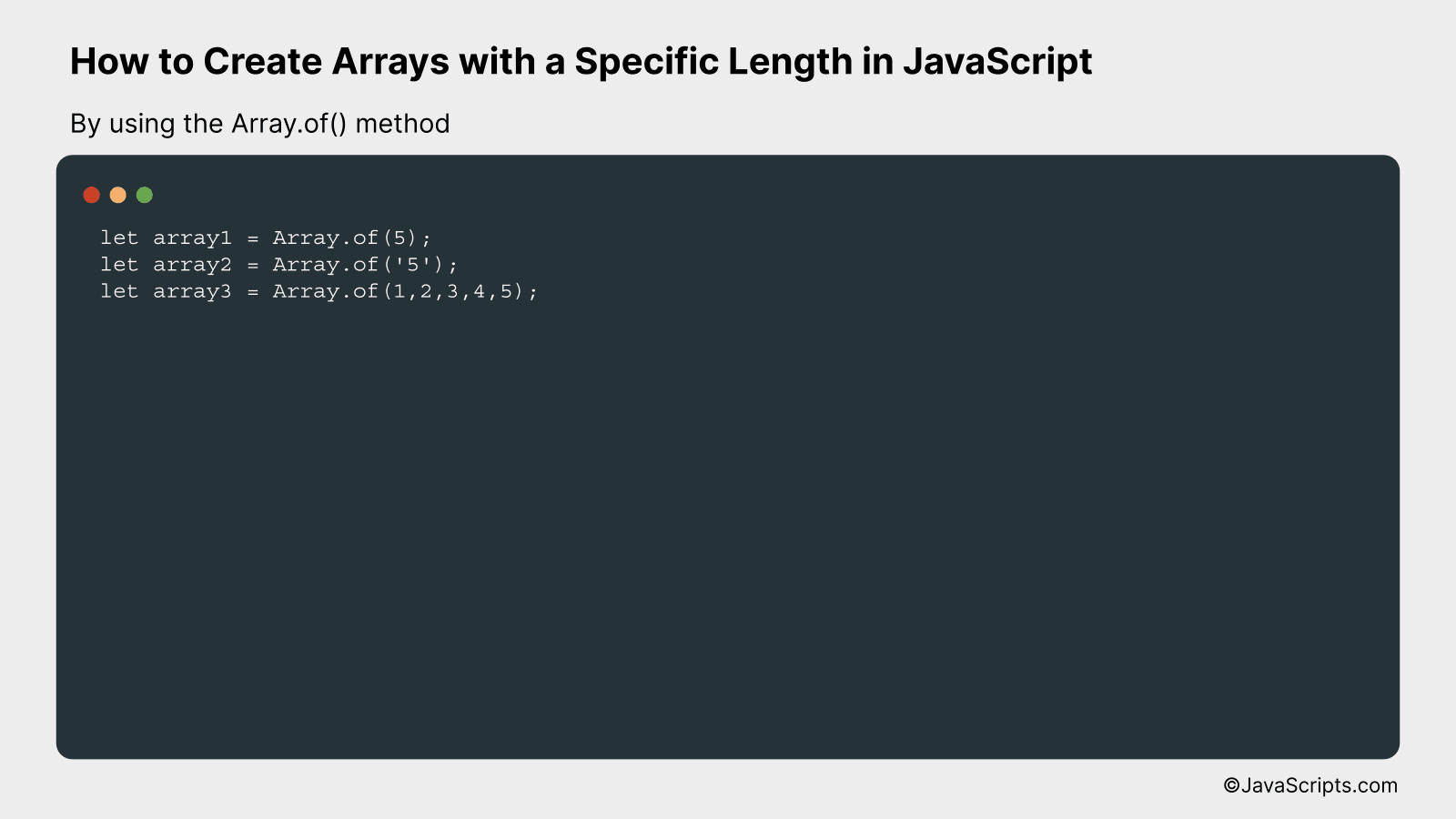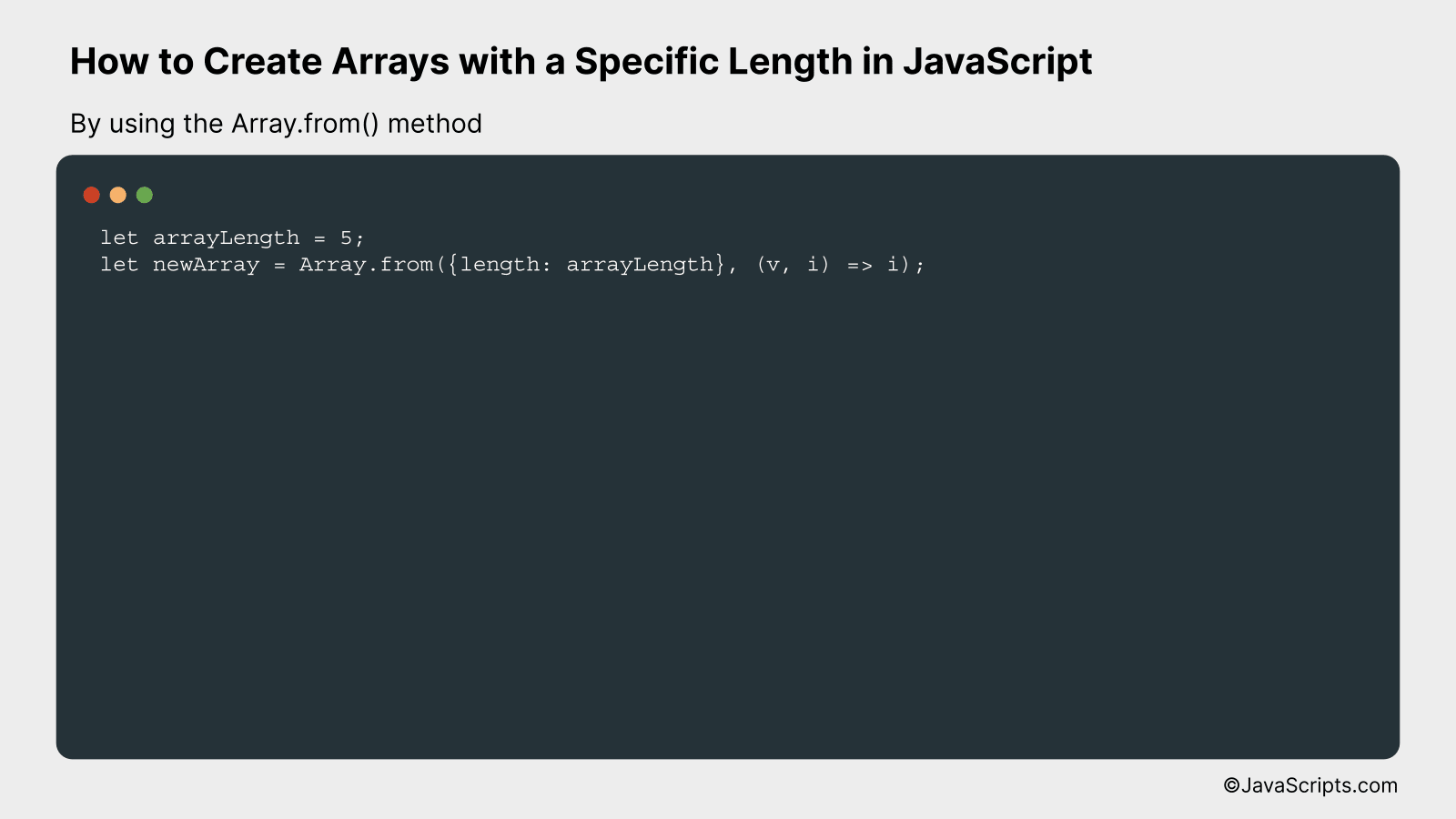# How to Create Arrays with a Specific Length in JavaScript

JavaScript is a powerful language at the heart of modern web development. Yet, one of the often overlooked elements in its toolbox is the humble array. Arrays are incredibly versatile and can make your code more efficient and easier to read.

As a JavaScript developer, you might sometimes need to create arrays of a specific length. This ability can prove crucial in dealing with larger datasets or when you need to perform certain operations a fixed number of times. We’ll explore how you can easily achieve this, using practical examples for clear understanding.

In this post, we will be looking at the following 3 ways to create arrays with a specific length in JavaScript:

• By using the Array() constructor
• By using the Array.of() method
• By using the Array.from() method

Let’s explore each…

## #1 – By using the Array() constructorIn JavaScript, you can create arrays of a specific length using the Array() constructor, which creates a new Array object with a specified number of undefined items. An example of how to create an array of length 5 is given below:

``````
let arr = new Array(5);
``````

### How it works

The ‘new Array()’ constructor in JavaScript creates an empty array with a length property set to the number of arguments provided during array creation. In our example, we’ve specified ‘5’ as the argument to create an array of length 5.

• Firstly, the ‘new’ keyword is used to create a new instance of an object. In our case, we’re creating a new instance of the Array object.
• Next, we call the Array() constructor. A constructor is a special method that’s used to initialize a newly created object. For the Array object, it accepts a number as an argument representing the desired length of the array.
• We provide ‘5’ as an argument to the Array() constructor, specifying that we want to create an array of length 5.
• Finally, the newly created array ‘arr’ has a length of 5, but contains no values – it is an array of 5 empty slots.

## #2 – By using the Array.of() methodThe Array.of() method in JavaScript creates a new Array instance with a variable number of arguments, regardless of number or type of the arguments. It’s a better way to create arrays with a single element or multiple elements. You can understand it better with the example given below:

``````
let array1 = Array.of(5);
let array2 = Array.of('5');
let array3 = Array.of(1,2,3,4,5);
``````

### How it works

The Array.of() method creates a new Array instance from a variable number of arguments, regardless of the number or type. In the case of passing a single argument, the method will still create an array with a single item, unlike the Array() constructor that would interpret the argument as the length of the array.

1. Array.of(5): Here, 5 is not the size of the array, but the first and only element. So, array1 will be .
2. Array.of(‘5’): Here, ‘5’ is not the size of the array, but the first and only element. So, array2 will be [‘5’].
3. Array.of(1,2,3,4,5): This creates an array with elements 1,2,3,4,5. So, array3 will be [1,2,3,4,5].

## #3 – By using the Array.from() methodThe Array.from() method in JavaScript creates a new array instance from an iterable or array-like object, which we can utilize to create arrays of a specific length. It is more clear when we explore this through an example:

``````
let arrayLength = 5;
let newArray = Array.from({length: arrayLength}, (v, i) => i);
``````

### How it works

The Array.from() method takes two arguments: the array-like or iterable object to convert to an array and a map function to call on every element of the array.

• First, we define a variable `arrayLength` to specify the desired length of the array.
• We then call `Array.from()` method and pass an object with a property of `length` which is set to the desired array length.
• The second argument to `Array.from()` is a map function, which is called on every element of the array. In this case, it’s an arrow function `(v, i) => i`, which simply returns the index, `i`, for each element.
• As a result, `newArray` will be an array of increasing numbers from 0 to `arrayLength - 1`.

Related:

In conclusion, creating arrays with a specific length in JavaScript is a straightforward task. Mastering this skill allows you to manipulate data more effectively, hence adding value to your coding journey.

Don’t forget to keep practicing because as the saying goes, practice makes perfect. Happy coding, and keep exploring the endless possibilities that JS arrays offer!